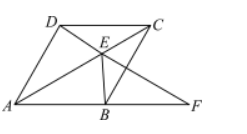. 如图，四边形 $A B C D$ 为菱形， $E$ 为对角线 $A C$ 上的一个动点（不与点 $A ， C$ 重合)，连接 $D E$ 并延长交射线 $A B$ 于点 $F$ ，连接 $B E$.
(1)求证: $\triangle D C E \cong \triangle B C E$ ；
(2)求证: $\angle A F D=\angle E B C$.【答案】 (1) 证明: :四边形 $A B C D$ 为菱形，
$$\therefore C D=B C ， \angle A C D=\angle A C B \text { ， }$$

\begin{aligned} & \left\{\begin{array}{l} C D=B C \\ \angle A C D=\angle A C B ， \\ C E=C E \end{array}\right. \\ & \therefore \triangle D C E \cong \triangle B C E(S A S) \text {; } \end{aligned}
(2) 证明: $\because \triangle D C E \cong \triangle B C E$ ，
$$\therefore \angle C D E=\angle E B C \text {, }$$
$\because$ 四边形 $A B C D$ 为菱形，
$\therefore A B \| C D$,
\begin{aligned} & \therefore \angle C D F=\angle A F D, \\ & \therefore \angle A F D=\angle E B C . \end{aligned}